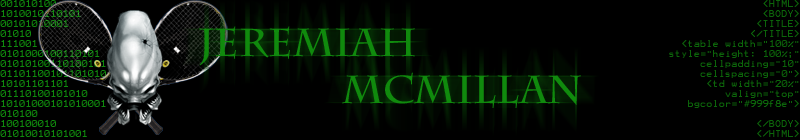# Variables

## What are variables

Think of variables as a box you can put values into

Variables are place holders for data. Data comes in the form on whole numbers, decimals, text, and objects.

## Data types in Java

String = Text data which is enclosed by double quotes " "

"Hello"

"Goodbye1"

"12345"

int = Integers are numbers wiithout decimals

123
34
55

double = Doubles are numbers with decimals

50.99
33.4433
123.2

## How to declare a variable format

To declare a variable, follow this format.

(data type) (variable name) = (value - optional)

Setting the value of a variable is optional

### Example

String name = "James"; //creates a string

int num1 = 9; //creates an integer

int num2; //creates an integer variable with no value
num2=23; // sets value to 23

double num3= 9.78; //creates a double

Student joeObj = new Student(ìJoe Schmoî , 16, 23432); //creates a new instance of a student class

## Concat variables - Appending Variables

You can concat a variable to String by using the plus + operator. If you concat a number type (double or int) to a String, it makes the data a String.

String fname = "Joe"; // name is a String
String lname = "Schmo"; // name is a String
int id=44523; //id is an integer

String fullIdName = fname + "--" id + "--" + lname;

System.out.println(fullIdName);

output
Joe--44532--Schmo

System.out.println("The id value is " + id);

output
The id value is 44532

## Variable Expressions - Making Calculations

You normally use numeric variables to make calculations.

### The + Operator

Use the + operator to add two ints together

int a;
int b;
a = 5 + 6; // a contains 11
b = a + 3; // b contains 14
a + b; // not allowed, does nothing
a = a + 1; // a contains 12, and b?

double a;
double b;
a = 3.14159; // a contains 3.14159
b = a + 2.1; // b contains 5.24159
a += 1.6; // shortcut for a = a + 1.6;
a++; // shortcut for a = a + 1.0;

## The - Operator

Exactly like + except performs subtraction

int a;
int b;
a = 5 - 6; // a contains -1
b = 3 - a; // b contains 4
a -= 10; // shortcut for a = a ñ 10;
a--; // shortcut for a = a ñ 1;

double a;
double b;
a = 3.14159;// a contains 3.14159
b = a - 2.1; // b contains 1.04159

### The * Operator

The * operator performs multiplication

int a;
int b;
a = 5 * 6; // a contains 30
b = a * 3; // b contains 90
a *= 2; // shortcut for a = a * 2;

double a;
double b;
a = 3.14159;// a contains 3.14159
a = b * 0.5; // a contains 0.520795

### The / Operator

The / operator performs integer division

Not the same as regular division

int a;
int b;
a = 3; // a contains 3
b = a / 2; // b contains 1
a /= 2; // shortcut for a = a / 2;

### The % Operator for int

The % operator is the mod operator
It finds the remainder after division

int a;
int b;
a = 8; // a contains 8
b = a % 5; // b contains 3
a %= 2; // shortcut for a = a % 2;

### Shortcuts

Some expressions are used so often, Java gives us a short cut
x = x + y; can be written x += y;
x = x + 1; can be written x++;

int x;
x = 6; // x contains 6
x += 4; // x contains 10
x++; // x contains 11

## Casting

You cannot directly store a double value into an int variable

int a = 2.6; // fails!

However, you can cast the double value to convert it into an int

int a = (int)2.6; // succeeds! (a = 2)

Casting tells the compiler you want the loss of precision to happen
You can always store an int into a double

## Rounding

In Java, the conversion of a double into an int does not use rounding
As in the case of integer division, the value is always rounded down

You can think of this as using the floor function from math

If you want to round normally, you can simply add 0.5 before the cast

## Data TypesCredit: A Slides are modified with permission from Barry Wittman at Elizabethtown College